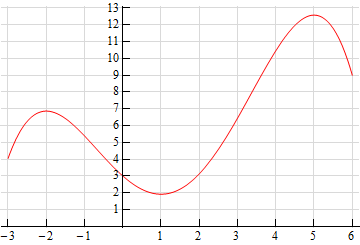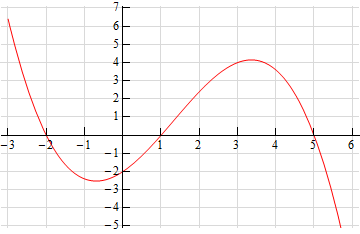Paul's Online Notes
Home / Calculus I / Derivatives / Interpretation of the Derivative
Show Mobile Notice Show All Notes Hide All Notes
Mobile Notice
You appear to be on a device with a "narrow" screen width (i.e. you are probably on a mobile phone). Due to the nature of the mathematics on this site it is best views in landscape mode. If your device is not in landscape mode many of the equations will run off the side of your device (should be able to scroll to see them) and some of the menu items will be cut off due to the narrow screen width.

### Section 3.2 : Interpretation of the Derivative

5. Below is the graph of some function, $$f\left( x \right)$$. Use this to sketch the graph of the derivative, $$f'\left( x \right)$$.Show All Steps Hide All Steps

Hint : Where will the derivative be zero? This gives us a couple of starting points for our sketch.
Start Solution

From the graph of the function it is pretty clear that we will have horizontal tangent lines at $$x = - 2$$, $$x = 1$$ and $$x = 5$$. Because we will have horizontal tangents here we also know that the derivative at these points must be zero. Therefore, we know the following derivative evaluations.

$f'\left( { - 2} \right) = 0\hspace{0.5in}f'\left( 1 \right) = 0\hspace{0.5in}f'\left( 5 \right) = 0$
Hint : Recall that the derivative can also be used to tell us where the function is increasing and decreasing. Knowing this we can use the graph to determine where the derivative will be positive and where it will be negative.
Show Step 2

The points we found above break the $$x$$-axis up into regions where the function is increasing and decreasing. Recall that if the derivative is positive then the function is increasing and likewise if the derivative is negative then the function is decreasing. Using these ideas we can easily identify the sign of the derivative on each of the regions. Doing this gives,

\begin{align*}\hspace{0.25in}x & < - 2 & \hspace{0.25in}f'\left( x \right) > 0\\ - 2 < x & < 1 & \hspace{0.25in}f'\left( x \right) < 0\\ 1 < x & < 5 & \hspace{0.25in}f'\left( x \right) > 0\\ x & > 5 & \hspace{0.25in}f'\left( x \right) < 0\end{align*}
Hint : At this point all we have to do is try and put all this together and come up with a sketch of the derivative.
Show Step 3

In the range $$x < - 2$$ we know the derivative must be positive and that it must be zero at $$x = - 2$$ so it makes sense that just to the left of $$x = - 2$$ the derivative must be decreasing.

In the range $$- 2 < x < 1$$we know that the derivative will be negative and that it will be zero at the endpoints of the range. So, to the right of $$x = - 2$$ the derivative will have to be decreasing (goes from zero to a negative number). Likewise, to the left of $$x = 1$$ the derivative will have to be increasing (goes from a negative number to zero).

Note that we don’t really know just how the derivative will behave everywhere in this range, but we can use the general behavior near the endpoints and go with the simplest way to connect the two up to get an idea of what the derivative should look like.

Following similar reasoning we can see that the derivative should be increasing just to the right of $$x = 1$$ (goes from zero to a positive number), decreasing just to the left of $$x = 5$$ (goes from a positive number to zero) and decreasing just to the right of $$x = 5$$ (goes from zero to a negative number).

Show Step 4

So, putting all of this together here is a sketch of the derivative. Note that we included the a scale on the vertical axis if you would like to try and estimate some specific values of the derivative as we did in Example 4 of this section.